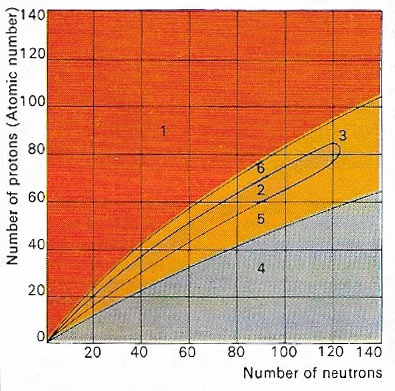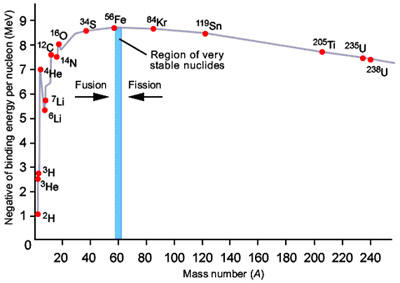A# atomic nucleusFig 1. The nucleus of an atom is made up of protons and neutrons. Only certain combinations of these subatomic particles are stable (2). Atomic nuclei containing a large excess of protons (1) or of neutrons (4) are both highly unstable. Beyond the stable region is a group of atoms (3) where nuclei break apart to form smaller, more stable nuclei. Below (5), and above (6), the stable regions are unstable nuclei, which decay into stable elements.Fig 2. Variation of binding energy with mass number.

The atomic nucleus is the center of an atom, around which orbits a cloud of electrons. The nucleus accounts for almost all of an atom's mass. It consists of protons and neutrons, collectively known as nucleons, which are bound bound together by the strong force. The number of protons in a nucleus is called its atomic number, while the number of nucleons is the mass number (Fig 1). Because protons are positively charged and neutrons have no charge, a nucleus has an overall positive charge; this is cancelled out, however, by the negatively charged electrons that orbit the nucleus. Under the right conditions, nuclei may undergo nuclear fission or fusion, with the release of large amounts of energy.

Following the discovery (1896) of radioactivity by Henri Becquerel, in 1911 Ernest Rutherford proposed the existence of the nucleus after identifying alpha and beta particles. For more, see Rutherford's experiment and atomic model.

## Binding energy

Binding energy is the amount of energy released when a particular isotope is formed (Fig 2). Protons and neutron are held together by the strong force, which only acts over very small distances but is able to overcome the electrostatic repulsion between protons. The strength of the bonding is measured by the binding energy per nucleon where "nucleon" is a collective name for neutrons and protons. The mass deficiency reflects the fact that the total mass of the nucleus is less than the sum of the mass of the individual neutrons and protons that formed it. The difference in mass is equivalent to the energy released in forming the nucleus.

The general decrease in binding energy beyond iron is due to the fact that, as nuclei gets bigger, the ability of the strong force to counteract the electrostatic repulsion between protons becomes weaker. The most tightly bound isotopes are 62Ni, 58Fe, and 56Fe, which have binding energies of 8.8 MeV per nucleon. Elements heavier than these isotopes can yield energy by nuclear fission; lighter isotopes can yield energy by fusion.

## Mass deficiency

Mass deficiency, also known as mass defect, is a term that describes the fact that nuclei, made up of protons and neutrons, have a smaller rest mass than the sum of the rest masses of the protons and neutrons forming the nucleus. The mass difference is equal to the released binding energy. For the alpha particle with a mass of 4.00151 atomic mass units, the structure of two protons (1.00728 atomic mass units each) and two neutrons (1.00866 atomic mass units each), a mass defect of 0.03037 atomic mass units results. This corresponds to an energy (binding energy) of about 28 million electronvolts (MeV).